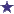# How Do I Calculate Pediatric Tetracycline Dosing by Weight?

by Rebecca
(Vero Beach, FL, USA)Pediatric Tetracycline Dosing by Weight

ORDER:TETRACYCLINE ELIXIR QID FOR A CHILD WEIGHING 50LB AT A DOSAGE OF 8MG/KG/DAY IN EQUALLY DIVIDED DOSES.

HOW MANY MG WOULD ONE DOSE CONTAIN?

TETRACYCLINE IS SUPPLIED AT 50MG PER 7ML. HOW MANY ML SHOULD BE ADMINISTERED?

### Comments for How Do I Calculate Pediatric Tetracycline Dosing by Weight?

Average RatingRatingDont give tetracycline to children 8 and under by: Anonymous Dont give tetracycline to children 8 and under

 RatingTetracycline math problem by: David Shedd The child weighs 50 pounds. Convert 50 pounds to kilograms = 22.67kg. 8mg/kg/day = 22.67kg times 8mg = 181.36mg/day. Divide 181.36mg by 4 (4 times a day) and you get 45.34mg per dose. One dose should contain 45.34mg of Tetracycline. If there are 50mg/7ml of Tetracycline Elixir, then you divide 45.34mg by 50 (50MG PER 7ML) = 0.906 x 7ml equals 6.34ML to be dispensed.How many ML's should be administered? That depends on how long the doctor wants the child to be on it. If it is a 10 day course, multiply 6.34ML by 4 = 25.39ML per day, multiplied by 10 = 253.9ML.Thanks for the question!